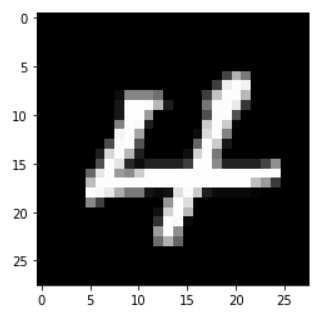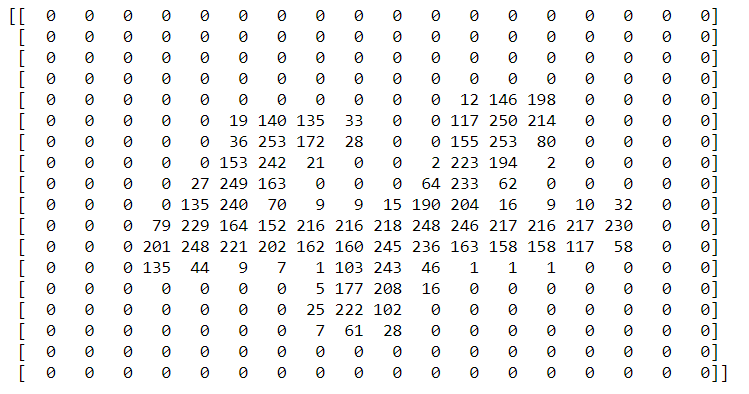# A Tip A Day – Python Tip #7: OpenCV – CV2: imread() and resize()

In this article we are going to learn some of the major use of OpenCV or CV2.

In this article we are going to learn some of the major use of OpenCV or CV2.

OpenCV is a library mainly used to read/write and manipulate images.

You can find the official page here https://pypi.org/project/opencv-python/.

Installation: pip install opencv-python

Importing Library: import cv2

Supported Versions: 3.6, 3.7, 3.8, 3.9

## Basic understanding of image:

We all know that an image is nothing but an array of pixels.
Each pixel is a number.

Based on the number the color of the pixel will be.

Example: 0 – Black and 1 – White.

Lets consider we have a small image of 28 X 28 pixels.

``````import cv2

image_path="4.png"

#Now we have the image in img variable.
#To visualize the image we can use matplotlib.pyplot

import matplotlib.pyplot as plt
plt.imshow(img, cmap="gray")
``````From the above program, you can see that we have a image “4.png”.

0 mentions the color of the image. 0 as second parameter will read the image in gray-scale mode. i.e. the image will only be a 2D array and the 3rd dimension for color will not be added.

Other available Flags are given below:

This image can be visualized using matplotlib.pyplot imshow(img). If we do not mention the cmap value, matplotlib will automatically assign a colormap to it.

The shape of this image can be found using the shape attribute as it is an array of pixels(again numbers).

``````img.shape
``````

(28, 28)

## Resize Image using cv2.resize()

Lets explore the values of the array.

The array shape is 2D 28 X 28. To have a good view of array in notebook result, I have resized the image to 18 X 18.

``````import numpy as np
number_arr = np.asarray(img)

img_resized = cv2.resize(img, (18,18))

print(np.asarray(img_resized))
``````You can see that there is a 4 shape in the array where numbers are > 0.

Interesting! isn’t it? Enjoy!

We will meet with a new tip in Python. Thank you! 👍

Like to support? Just click the heart icon ❤️.

Happy Programming!🎈.##### Asha Ponraj

Data science and Machine Learning enthusiast | Software Developer | Blog Writter

Articles: 86